# Soup from canteen

For how many people is 90 liters of soup enough if we assume 3/8 liter of soup per person in the canteen?

Result

n =  240

#### Solution:Leave us a comment of example and its solution (i.e. if it is still somewhat unclear...):

Showing 0 comments:Be the first to comment!#### To solve this verbal math problem are needed these knowledge from mathematics:

Need help calculate sum, simplify or multiply fractions? Try our fraction calculator. Tip: Our volume units converter will help you with converion of volume units.

## Next similar examples:

1. Percent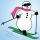From 99 children who participated in the ski course were 23 excellent skiers, 13 good and 20 average and the rest were beginners. Calculate this data in percentages.
2. TVsProduction of television sets increased from 3,500 units to 4,200 units. Calculate the percentage of production increase.
3. Milk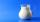At the kindergarten, every child got 1/5 liter of milk in the morning and another 1/8 liter of milk in the afternoon. How many liters were consumed per day for 20 children?
4. Vehicle tankA vehicle tank was 3/5 full of petrol. When 21 liters of fuel was added it was 5/6 full. How many liters of petrol can the tank hold?
5. Container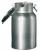How many three-quarters liters jar fill 30 liters container?
6. Lemonade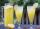How many 1/4 cup servings are in 2 and 1/3 cups of lemonade?
7. Cleaning windowsCleaning company has to wash all the windows of the school. The first day washes one-sixth of the windows of the school, the next day three more windows than the first day and the remaining 18 windows washes on the third day. Calculate how many windows ha
8. Bag of peanuts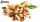Joe eat 1/3 of a bag of peanuts, mark eat 1/4 of the remaining in the bag of peanuts, Alvin eat 1/2 of the remaining bag of peanuts, peter eat 10 peanuts, there are 71 peanuts left. Hon many peanuts were in the bags?
9. Sewing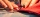Beth's mother can sew 235 pairs of short pants in 6 days while Lourdes can sew 187 pairs in 8 days. How many more pairs of short pants can Beth's mother sew?
10. Find the 11Find the quotient of 229.12 and 12.32
11. Percent calculationCalculate 61% if 85% is 38.
12. Equation with xSolve the following equation: 2x- (8x + 1) - (x + 2) / 5 = 9
13. Equation with fractionsSolve equation: ? It is equation with fractions.
14. Typing course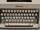Before taking a typing course, Terrence could type 39 words per minute. By the end of the course, he was able to type 68 words per minute. Find the percent increase.
15. FractionsThree-quarters of an unknown number are 4/5. What is 5/6 of this unknown number?
16. InqualitySolve inequality: 3x + 6 > 14
17. UN 1If we add to an unknown number his quarter, we get 210. Identify unknown number.